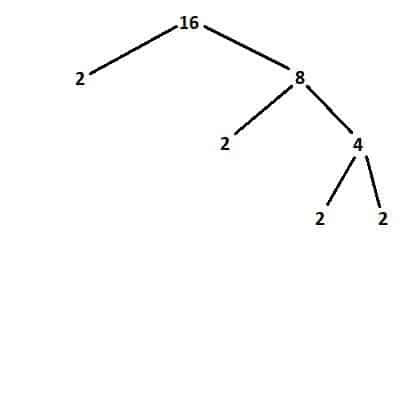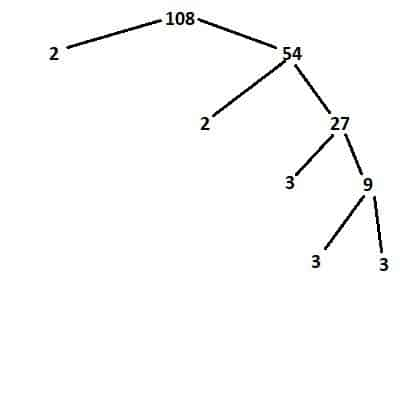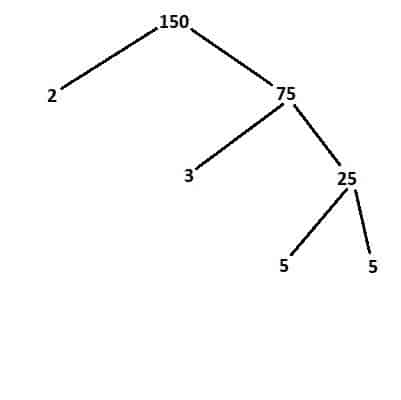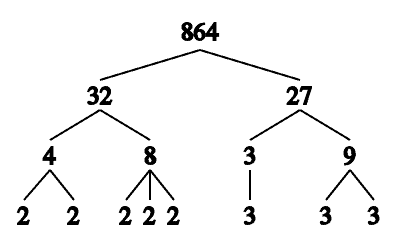# Factors Of A Number (5 Common Questions Answered)

Factoring numbers helps us to understand prime numbers, and it is also important in algebra (for factoring, among other uses).

So, what are the factors of a number?  A factor F of a whole number N satisfies the equation N = FX, where F and X are whole numbers. We also say that N is divisible by F. If F is a prime number, then we say that F is a prime factor of N. We can find small prime factors of a number with some basic divisibility rules.

Once we factor a number, we can figure out how many distinct factors it has.

Let’s get started.

## Factors Of A Number

For a whole number N, a factor F satisfies the equation

• N = FX

where F and X are whole numbers.This factor tree shows all factors and prime factors of 16. The only prime factor is 2 (with multiplicity 4), and the factors are 1, 2, 4, 8, and 16.

We can also say that N is divisible by F (F is a divisor of N).

A factor F need not be prime, but if F is a prime number, we say that F is a prime factor of N. We can find the smaller prime factors (such as 2, 3, and 5) of a number with some basic divisibility rules (more on this later).

There are always more factors of a number than there are prime factors.  This is because every prime factor is a factor, but not every factor is a prime factor.

For example, 1 is a factor of every number, but 1 is not prime, so 1 is not a prime factor.

The factors of a number N are numbers we can multiply together to get a product of N.  Remember that prime factorizations are unique (according to the Fundamental Theorem of Arithmetic).  However, there may be more than one way to write a number in terms of its factors.Here we see the factor tree for 108. The prime factors are 2 (multiplicity 2) and 3 (multiplicity 3), meaning there are 12 factors.

For example, the prime factorization of 12 is 2*2*3, which is unique.  However, we can write 12 = 1*12, 12 = 2*6, and also 12 = 3*4, which are three different factorizations (but all are equivalent to 2*2*2 when we reduce to prime factors).

We need to know which factorization to use, depending on the context.  In algebra, we would factor quadratics as follows by using the factorizations above:

• x2 + 13x + 12 = (x + 1)(x + 12)
• x2 + 8x + 12 = (x + 2)(x + 6)
• x2 + 7x + 12 = (x + 3)(x + 4)

### Can Factors Of A Number Be Negative?

Factors of a number can be negative.  This is useful for factoring quadratics in algebra.  For example:

• x2 – 13x + 12 = (x – 1)(x – 12)
• x2 – 8x + 12 = (x – 2)(x – 6)
• x2 – 7x + 12 = (x – 3)(x – 4)

These are similar to the factorizations as above, but with a negative sign switched on the left side.

However, it is important to remember that prime factors of a number cannot be negative.  By definition, a prime number is positive, so there are no negative prime factors.

### What Is The Difference Between Multiples & Factors Of A Number?

Remember that a multiple of a number N has the form M = KN, where K and M are whole numbers.

As mentioned earlier, a factor F of a number M satisfies the equation N = FX, where F and X are whole numbers.

Also, a number N has infinitely many multiples (N, 2N, 3N, 4N, …), but only finitely many factors (since the positive factors are whole numbers between 1 and N).

In addition, a positive multiple of N is greater than or equal to N, while a positive factor of N is between 1 and N.

## How To Find Factors Of A Number

We can do trial and error to find factors of a number by hand.  However, it will save time to use a computer to do this type of checking.

There are also some shortcuts for finding small factors of a number by hand.  For example:

• Any even number (ending in 0, 2, 4, 6, or 8) has a prime factor of 2.
• Any number whose digits add up to a multiple of 3 has a prime factor of 3.
• Any number ending in 0 or 5 has a prime factor of 5.

We can combine the rules for 2 and 5 to say that any number ending in 0 has a factor of 10 (the product of 2 and 5).

If a number ends in K zeros, then it has at least K 2’s and at least K 5’s in its prime factorization.This is the factor tree for 150. Since it ends in 0, it has a factor of 10, which means prime factors of 2 and 5.

### What Is The Easiest Way To Find The Factors Of A Number?

The easiest way to find the factors of a number is to start off by factoring out the smallest primes as many times as possible.  Then, we will have the prime factorization (we can also use a factor tree to visualize this).

After that, we can combine the primes as products to get all possible combinations (factors).This is the factor three for 864. It has prime factors of 2 (multiplicity 5) and 3 (multiplicity 3), meaning there are 24 factors.

For example, let’s take the number 180.

We’ll start with the “ends in 0” rule.  This means that 10 is a factor of 180, so 2 and 5 are factors.

So we can reduce to 180 = 18*10 or 18*2*5.

Also, 18 is even, so we can factor it as 18 = 2*9.

Now, 9 is a perfect square: 9 = 3*3.

This reduces everything to prime factors:

• 180
• =18*10
• =(2*9)*10
• =(2*3*3)*10
• =(2*3*3)*(2*5)
• =22*32*5

To find the factors, we write out all possible products of the prime factors (and also 1, which is always a factor):

• 1
• 2
• 3
• 4 = 22
• 5
• 6 = 2*3
• 9 = 32
• 10 = 2*5
• 12 = 22*3
• 15 = 3*5
• 18 = 2*32
• 20 = 22*5
• 30 = 2*3*5
• 36 = 22*32
• 45 = 32*5
• 60 = 22*3*5
• 90 = 2*32*5
• 180 = 22*32*5

### How Many Factors Does A Number Have?

We can use the prime factorization of a number to find out how many factors it has.

Let’s say that the prime factorization of N is:

• N = Πpixi (from I = 1 to k)

where pi is a prime factor (all pi are unique), ni is the multiplicity of pi, and k is the number of unique prime factors of N.

Then the number of factors of N is (x1 + 1)*(x2 + 1)*…*(xk + 1).  This is because when we take products of prime factors, we have xi + 1 choices for each exponent: any exponent from 0 to xi.

We can use this formula to verify various scenarios.

For example, 1 factors as 20*30*… (that is, the exponent of every prime is 0).

Then (x1 + 1)*(x2 + 1)*…*(xk + 1) = (0 + 1)*(0 + 1)*…*(0 + 1) = 1*1*…*1 = 1.

Thus, the number 1 has only 1 factor: 1.

As another example, the prime number P has a prime factorization of P = P1 (the exponent of the only prime factor P is 1).

Then (x1 + 1)*(x2 + 1)*…*(xk + 1) = (1 + 1) = 2.

Thus, a prime number P has only two factors: 1 and P (this is also true by definition of a prime number).

Any other number N (where N is composite) has at least 3 factors: 1, F, and N, where F is not 1 or N.

Since N is composite, N either has a single prime factor with a multiplicity of at least 2, or it has at least 2 distinct prime factors.

In the first case, the number of factors is (x1 + 1) >= (2 + 1)= 3.

In the second case, the number of factors is at least (x1 + 1)(x2 + 2) >= (1 + 1)*(1 + 1) = 2*2 = 4.

### Can Excel Find Factors Of A Number?

There is no standard function in Excel that can find factors of a number.  However, you could write custom code in VBA to find the factors.

The best way would be to write a “helper” function that tells you whether a number is prime or not.  Then, pull out and save prime factors until what remains is prime.

Then, use the formula above to find the number of factors, and then list them all out.

### Example 1: What Are The Factors Of 6?

The prime factors of 6 are 2 and 3.

So, there are 4 factors of 6, which are 1, 2, 3, and 6.

### Example 2: What Are The Factors Of 12?

The prime factors of 12 are 2, 2, and 3.

So, there are 6 factors of 12, which are 1, 2, 3, 4, 6, and 12.

### Example 3: What Are The Factors Of 20?

The prime factors of 20 are 2, 2, and 5.

So, there are 6 factors of 20, which are 1, 2, 4, 5, 10, and 20.

### Example 4: What Are The Factors Of 24?

The prime factors of 24 are 2, 2, 2, and 3.

So, there are 8 factors of 24, which are 1, 2, 3, 4, 6, 8, 12, and 24.

### Example 5: What Are The Factors Of 50?

The prime factors of 50 are 2, 5, and 5.

So, there are 6 factors of 50, which are 1, 2, 5, 10, 25, and 50.

### Example 6: What Are The Factors Of 120?

The prime factors of 120 are 2, 2, 2, 3, and 5.

So, there are 16 factors of 120, which are 1, 2, 3, 4, 5, 6, 8, 10, 12, 15, 20, 24, 30, 40, 60, and 120.

## Conclusion

Now you know what the factors of a number are and how to find them.